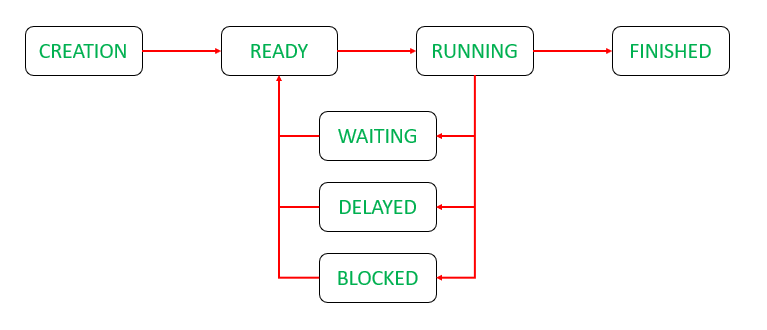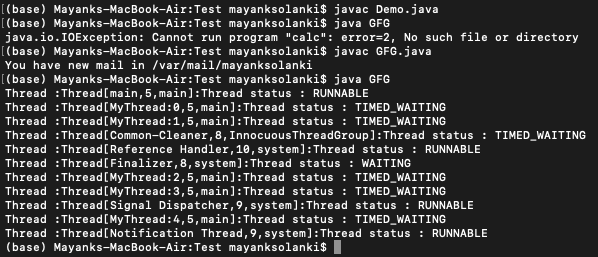# How to Display all Threads Status in Java?

• Last Updated : 24 Feb, 2022

Threads are light-weight processes within a process.. Multithreading in java is a feature that allows concurrent execution of two or more parts of a program to maximize the utilization of CPU. here the approach to retrieve the state of the thread is via getState() method of the Thread class. A java thread can exist in any one of the following states, the status of a thread is the state in which it exists at a given instance. The life cycle of a thread as shown above is the best way out to learn more about the states where the states are as follows:

• New
• Runnable
• Blocked
• Waiting
• Timed Waiting
• TerminatedNote: When a thread is getting executed all other threads are in blocking state and not in waiting state.

1. Threads are created by implementing the runnable interface.
2. The status of a thread can be retrieved by getState() method of the Thread class object.

Example:

## Java

 `// Java Program to Display all Threads Status` `// Importing Set class from java.util package``import` `java.util.Set;` `// Class 1``// helper Class implementing Runnable interface``class` `MyThread ``implements` `Runnable {` `    ``// run() method whenever thread is invoked``    ``public` `void` `run()``    ``{` `        ``// Try block to check for exceptions``        ``try` `{` `            ``// making thread to``            ``Thread.sleep(``2000``);``        ``}` `        ``// Catch block to handle the exceptions``        ``catch` `(Exception err) {` `            ``// Print the exception``            ``System.out.println(err);``        ``}``    ``}``}` `// Class 2``// Main Class to check thread status``public` `class` `GFG {` `    ``// Main driver method``    ``public` `static` `void` `main(String args[]) ``throws` `Exception``    ``{` `        ``// Iterating to create multiple threads``        ``// Customly creating 5 threads``        ``for` `(``int` `thread_num = ``0``; thread_num < ``5``;``             ``thread_num++) {` `            ``// Creating single thread object``            ``Thread t = ``new` `Thread(``new` `MyThread());` `            ``// Setting name of the particular thread``            ``// using setName() method``            ``t.setName(``"MyThread:"` `+ thread_num);` `            ``// Starting the current thread``            ``// using start() method``            ``t.start();``        ``}` `        ``// Creating set object to hold all the threads where``        ``// Thread.getAllStackTraces().keySet() returns``        ``// all threads including application threads and``        ``// system threads``        ``Set threadSet``            ``= Thread.getAllStackTraces().keySet();` `        ``// Now, for loop is used to iterate through the``        ``// threadset``        ``for` `(Thread t : threadSet) {` `            ``// Printing the thread status using getState()``            ``// method``            ``System.out.println(``"Thread :"` `+ t + ``":"``                               ``+ ``"Thread status : "``                               ``+ t.getState());``        ``}``    ``}``}`

Output:My Personal Notes arrow_drop_up